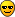# Dividing RooDataHist by imported ROOT TH1F

Hi,

I am trying to apply a trigger correction to my pT spectra. I have created the trigger efficiency as a TH1F in a ROOT file. What I want to do is import this histogram and divide my RooDataSet (with RooRealVar pT) with it. Importing is ok, but the trouble is converting the RooDataSet into a TH1F so that I may call TH1::Divide() and convert this histo back into a RooDataHist. Any ideas? Thanks in advance.

TH1F* trigSet = (TH1F*) gDirectory->Get(“hptTrigEF”) ;
TH1* dataSet2 = dataSet->createHistogram(“muonPt”,muonPt,Binning(86,4,90));
TH1F* dataSetCorr0 = new TH1F(“dataSetCorr”,“dataSetCorr”,86,4,ptmax);
dataSetCorr0->Divide(dataSet2,trigSet);
RooRealVar muonPtCorr(“muonPtCorr”,“p_{T}”,0,100,“GeV”);
RooDataHist* dataSetCorr = new RooDataHist(“dataSetCorr”,“dataSetCorr”,muonPtCorr,dataSetCorr0) ;
dataSetCorr->plotOn(framePt, RefreshNorm(), Binning(b1), DrawOption(“p”), MarkerSize(0.);

Better yet, is there a method to simply divide 2 RooDataHist objects? The ::Divide method doesn’t seem to work as it does with TH1X objects.

Hello,

What I usually do to create a histo brom a RooDataSet (although there might be a more elegant solution to it) is:

TTree* tree = dataSet->tree();
tree.Draw(“variable>>histo”);

What would however be better in this case would be to apply a per-event weight based on the trigger correction in RooFit’s dataset. See:

root.cern.ch/root/html/tutorials … vts.C.html

Regards,

– Gregory

Hi Gregory,

Thanks for the help. So if I perform the weighting procedure, I could fit the trigger efficiency to a Fermi function, which would allow for a parameterization that may be used in place of (x*x+10) in the weighting function argument:

// Construct formula to calculate (fake) weight for events
RooFormulaVar wFunc(“w”,“event weight”,"(x*x+10)",x) ;

but how do I obtain this parameterization using RooFit?

Hi Thomas,

If you want to make a likelihood fit for an efficiency, should should use both the
accepted and rejected data to construct the likelihood, rather than dividing things
and using approximate error bars (which in any case can only be used in a chiˆ2 fit
and not a likelihood fit). A complete example on how to do this is provided here

root.cern.ch/root/html/tutorials … fit.C.html

Wouter